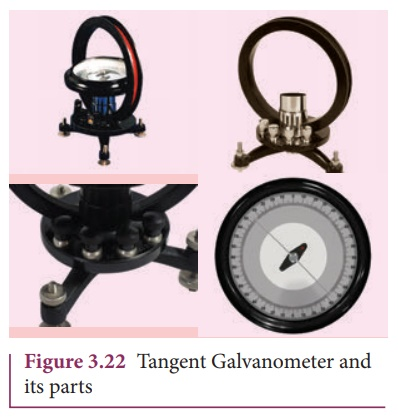Home | | Physics 12th Std | Tangent law and Tangent Galvanometer

# Tangent law and Tangent Galvanometer

Tangent Galvanometer is a device used to measure very small currents.

Tangent law and Tangent Galvanometer

Tangent Galvanometer (Figure 3.21) is a device used to measure very small currents. It is a moving magnet type galvanometer. Its working is based on tangent law.## Tangent law

When a magnetic needle or magnet is freely suspended in two mutually perpendicular uniform magnetic fields, it will come to rest in the direction of the resultant of the two fields.

Let B be the magnetic field produced by passing current through the coil of the tangent Galvanometer and BH be the horizontal component of earth’s magnetic field. Under the action of two magnetic fields, the needle comes to rest making angle θ with BH, such that## Construction

Tangent Galvanometer (TG) consists of copper coil wounded on a non-magnetic circular frame. The frame is made up of brass or wood which is mounted vertically on a horizontal base table (turn table) with three levelling screws as shown in Figure 3.22. The TG is provided with two or more coils of different number of turns. Most of the equipment we use in laboratory consists of 2 turns, 5 turns and 50 turns which are of different thickness and are used for measuring currents of different strengths.

At the center of turn table, a small upright projection is seen on which compass box (also known as magnetometre box) is placed. Compass box consists of a small magnetic needle which is pivoted at the center, such that arrangement shows the center of both magnetic needle and circular coil exactly coincide. A thin aluminium pointer is attached to the magnetic needle normally and moves over circular scale. The circular scale is divided into four quadrants and graduated in degrees which are used to measure the deflection of aluminium pointer on a circular degree scale. In order to avoid parallax error in measurement, a mirror is placed below the aluminium pointer.## Precautions

1.     All the nearby magnets and magnetic materials are kept away from the instrument.

2. Using spirit level, the levelling screws at the base are adjustedso that the small magnetic needle is exactly horizontal and also coil (mounted on the frame) is exactly vertical.

3. The plane of the coil is kept parallel to the small magnetic needle by rotating the coil about its vertical axis. So, the coil remains in magnetic meridian.4. The compass box (as shown in Figure 3.23) is rotated such that the pointer reads 0º – 0 º

## Theory

The circuit connection for Tangent Galvanometer (TG) experiment is shown in Figure 3.24. When no current is passed through the coil, the small magnetic needle lies along horizontal component of Earth’s magnetic field. When the circuit is switched ON, the electric current will pass through the circular coil and produce magnetic field. The magnetic field produced due to the circulatory electric current is discussed (in section 3.8.3). Now there are two fields which are acting mutually perpendicular to each other. They are:

(1) the magnetic field (B) due to the electric current in the coil acting normal to the plane of the coil.

(2) the horizontal component of Earth’s magnetic field (BH)Because of these crossed fields, the pivoted magnetic needle deflects through an angle θ. From tangent law (equation 3.29),

B = BH tan θ

When an electric current is passed through a circular coil of radius R having N turns, the magnitude of magnetic field at the center isFrom equation (3.29)  and equation (3.30), we getThe horizontal component of Earth’s magnetic field can be determined asEXAMPLE 3.9

A coil of a tangent galvanometer of diametre 0.24 m has 100 turns. If the horizontal component of Earth’s magnetic field is 25 × 10-6 T then, calculate the current which gives a deflection of 60º.

Solution

The diameter of the coil is 0.24 m.

Therefore, radius of the coil is 0.12 m.

Number of turns is 100 turns. Earth’s magnetic field is 25 x 10-6 T

Deflection is

θ=60 º  tan 60 º = √3 = 1.732I = 0.082 A

Tags : Construction, Precautions, Theory, Solved Example Problems , 12th Physics : Magnetism and Magnetic Effects of Electric Current
Study Material, Lecturing Notes, Assignment, Reference, Wiki description explanation, brief detail
12th Physics : Magnetism and Magnetic Effects of Electric Current : Tangent law and Tangent Galvanometer | Construction, Precautions, Theory, Solved Example Problems